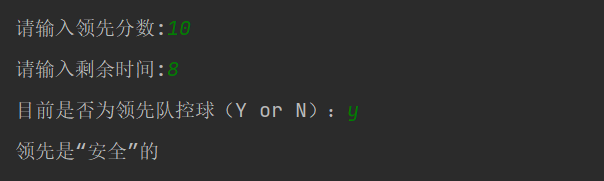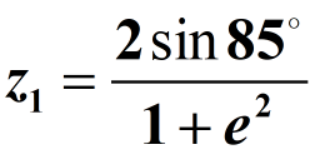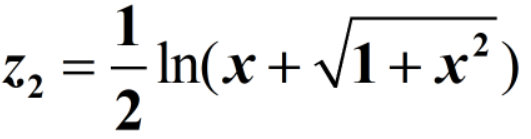#### 1. 输入一个百分制成绩，要求输出成绩等级A、B、C、D、E，其中`90~100分`为A，`80~89分`为B，`70~79分`为C，`60~69`分为D，60分以下为E。

• 用if语句实现；
• 输入百分制成绩后要判断该成绩的合理性，对不合理的成绩应输出出错信息。

``````a=eval(input('请输入成绩：'))
if a<0 or a>100 or a%1!=0:
print('您输入的成绩不合理，请检查后重新输入')
elif 90<=a<=100:
print('成绩等级:A')
elif 80<=a<=89:
print('成绩等级:B')
elif 70<=a<=79:
print('成绩等级:C')
elif 60<=a<=69:
print('成绩等级:D')
else:
print('成绩等级:E')
``````#### 2. 篮球比赛是高分的比赛，领先优势可能很快被反超。作为观众，希望能在球赛即将结束时，就提早知道领先是否不可超越。体育作家Bill James发明了一种算法，用于判断领先是否“安全”。

• 获取领先的分数 ，减去3分；

• 如果目前是领先队控球，则加0.5；否则减0.5（数字小于0则变成0）；

• 计算平方后的结果；

• 如果得到的结果比当前比赛剩余时间的秒数大，则领先是“安全”的。

``````'''

'''
time=eval(input('请输入剩余时间:'))
w=input('目前是否为领先队控球（Y or N）：')
if w == 'Y' or w == 'y':
g=(t+0.5)**2
else:
g=t-0.5
if g<=0:
g=0
g=g**2
if g>time:
print('领先是“安全”的')
else:
print('领先是“不安全”的')
``````#### 3.根据`y=1+3-1+3-1+……+(2n-1)-1`，求：

• y<3时的最大n值。

• 与（1）的n值对应的y值。

代码：

``````x=1
y=0
while y<3:
y=y+1/(2*x-1)
x=x+1
print('y<3时的最大n值为{}'.format(x-1))
print('与(1)的n值对应的y值为{}'.format(y-1/(2*x-1)))
``````

``````y<3时的最大n值为57

``````

#### 4. 小明单位发了100元的购物卡，小明到超市买三类洗化用品：洗发水（15元）、香皂（2元）、牙刷（5元）。要把100元正好花掉，可有哪些购买组合？

``````'''

'''
money=100
n=money//15
for i in range(n,-1,-1):
m=(money-i*15)//5
for j in range(m,-1,-1):
k=(money-i*15-j*5)//2
if (money-i*15-j*5)%2==0:
print('可选择的购买组合:\t\t购买洗发水 {} 瓶，香皂 {} 块，牙刷 {}个。'.format(i,j,k))
``````

``````可选择的购买组合:		购买洗发水 6 瓶，香皂 2 块，牙刷 0个。

``````

#### 5. 设计一个猜数游戏。首先由计算机产生一个[1,100]之间的随机整数，然后由用户猜测所产生的随机数。根据用户猜测的情况给出不同提示，如猜测的数大于产生的数，则显示“High”，小于则显示“Low”，等于则显示“You won !”，游戏结束。用户最多可以猜7次，如果7次均未猜中，则显示“You lost !”，并给出正确答案，游戏结束。游戏结束后，询问用户是否继续游戏，选择“Y”则开始一轮新的猜数游戏；选择“N”则退出游戏。

``````chose = 'y'
while chose=='Y' or chose=='y':
import random
num = random.randint(1,100)
def judge(b):
if b == num:
return 1
else:
return 0
for i in range(1,8):
b=eval(input('请输入您第{}次所猜的整数：'.format(i)))
if judge(b)==1:
print("You won !")
break
elif b > num:
print("high")
elif b < num:
print("low")
if judge(b)==0:
print("You lost !")
chose=input('请输入Y(y)继续进行游戏，N(n)退出游戏：')
while chose != 'Y' and chose != 'y' and chose != 'N' and chose != 'n':
print('输入有误，请重新输入Y(y)继续进行游戏，N(n)退出游戏：',end = '')
chose=input()
``````

``````请输入您第1次所猜的整数：2
low

low

low

low

low

low

high
You lost !

``````

#### 6. 建立1个包含10个字符的字符串，并根据键盘输入的数字n输出字符串中的第n个字符。当n值超过字符串的索引时，自动转为输出字符串中的最后1个字符。

``````'''

'''
n=int(input('请输入数字n：'))
a='pengyuanyu'
try:
print(a[n-1])
except:
print(a)
``````

``````请输入数字n：5
y
``````

#### 7. 编写函数，该函数可以输入任意多个数，函数返回输出所有输入参数的最大值、最小值和平均值。

``````'''

'''
import numpy as py
x=input('请输入一组数并用空格隔开：')
def f(x):
lis =list(x.split(' '))
for i in range(len(lis)):
lis[i]=eval(lis[i])
print('该组数值的最大值为：',max(lis))
print('该组数值的最小值为：',min(lis))
print('该组数值的平均值为：',py.mean(lis))
f(x)
``````

``````请输入一组数并用空格隔开：5 4 10

``````

#### 8. 一个人赶着鸭子去每个村庄卖，每经过一个村子卖去所赶鸭子的一半又一只。这样他经过了七个村子后还剩两只鸭子，问他出发时共赶多少只鸭子？

``````def f(n):
if n == 8:
return 2
else:
sum = f(n+1)*2+2
return sum
print('一共有{}只鸭子'.format(f(1)))
``````

``````一共有510只鸭子
``````

#### 9.将复数2.3×103-1.34×10-3j赋值给变量A，并分别提取A的实部和虚部。

``````>>> A=complex(2.3e3,-1.34e-3)
>>> print(A.real)
2300.0
>>> print(A.imag)
-0.00134
``````

#### 10.计算下列表达式的值：``````import math
z1=(2*(math.sin(math.pi*85/180)))/(1+math.e**2)
print(z1)
``````

``````0.23749863783095462
````````````import math
x=int(input('请输入整数：'))
z2=1/2*math.log(x+math.sqrt(1+math.pow(x, 2)))
print(z2)
``````

``````请输入整数：5
1.1562191706363762
``````

#### 11.建立一个包含10个字符的字符串A，然后对该字符串进行如下操作：

（1）计算输出字符串的长度；

（2）从第1个字符开始，每间隔2个字符取1个字符，组成子字符串B；

（3）将字符串A倒过来重新排列产生新的字符串C；

（4）将字符串A的前4个字符与字符串C的后5个字符进行组合，产生字符串D。

`````` A=input()
>>> len(A)
>>> B=print(A[::3])
>>> C=print(A[::-1])
>>> D=print(A[:3]+C[-5:])
``````

#### 12.分别格式化输出0.002178对应的科学表示法形式、具有4位小数精度的浮点数形式和百分数形式，并将输出宽度设定为10、居中对齐、星号*填充。

``````x=0.002178
print("x对应的科学表示法形式为:",("%e"%x).center(10,'*'))
print('x具有4位小数精度的浮点数形式为：',('{0:.4f}'.format(x)).center(10,'*'))
print('x百分数形式为:',(('{0:.2f}%'.format(x*100)).center(10,'*')))
``````

``````x对应的科学表示法形式为: 2.178000e-03
x具有4位小数精度的浮点数形式为： **0.0022**
x百分数形式为: **0.22%***
``````

#### 13.编写程序，从键盘输入一个1~7的数字，格式化输出对应数字的星期字符串名称。如：输入3，返回“您输入的是星期三”。

``````n =int(input('输入一个1~7的数字:'))
if(n==1):
print("您输入的是星期一")
elif(n==2):
print("您输入的是星期二")
elif(n==3):
print("您输入的是星期三")
elif(n==4):
print("您输入的是星期四")
elif(n==5):
print("您输入的是星期五")
elif(n==6):
print("您输入的是星期六")
elif(n==7):
print("您输入的是星期日")
``````

``````输入一个1~7的数字3

``````

#### 14.数字加密游戏：编程程序，从键盘任意输入1个4位数，将该数字中的每位数与7相乘，然后取乘积结果的个位数对该数字进行替换，最后得到1个新的4位数。

``````n = int(input('任意输入1个4位数:'))
if 1000<=n<=9999:
a=n%10
b=(n-a)%100/10
c=(n-a-10*b)%1000/100
d=(n-a-10*b-100*c)%10000/1000
a=a*7%10
b=b*7%10
c=c*7%10
d=d*7%10
n=1000*d+100*c+10*b+a
print(int(n))
elif n<=1000 or n>=9999:
print("您输入的数字不符合要求，请输入一个四位数字")
``````

``````任意输入1个4位数:111

2222
``````

Python基础入门教程推荐：更多Python视频教程-关注B站：Python学习者
https://www.bilibili.com/video/BV1LL4y1h7ny?share_source=copy_web

Python爬虫案例教程推荐：更多Python视频教程-关注B站：Python学习者
https://www.bilibili.com/video/BV1QZ4y1N7YA?share_source=copy_web